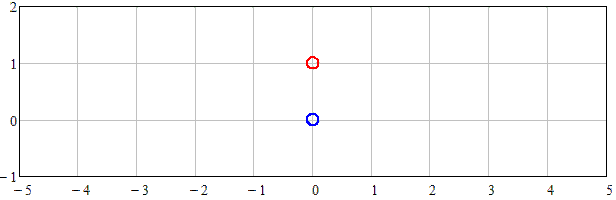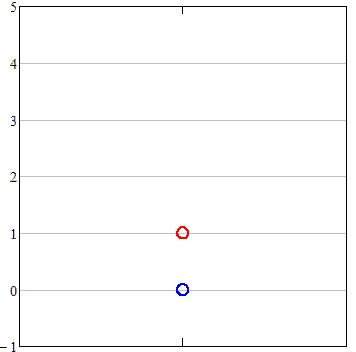Research website of Vyacheslav Gorchilin
2019-10-02
The conversion unit in the vector
The unusual name of this work is associated with non-traditional notions of space, and everything located in it, the world around us. In the first part we will show and prove that a scalar is a rolled vector, and discover how from the first "cook" the second, and Vice versa. This approach to things will allow a new look at the concept of measures and dimensions — length, mass, speed, acceleration, etc., which has long been well established in our minds. The work is interesting as classical physicists and netradiciniu, and some of its conclusions would relate to our esteemed and tireless seekers of free energy :)
1. Two points
Figure (1) shows the motion of two points relative to each other. Here, on the horizontal axis delayed values of the coordinates $$x$$ and the vertical coordinate value $$y$$. Under the "points" we mean the geometric points that have no dimensions , but in the figures for better clarity, we will depict it in small circles.Fig.1. The motion of one point relative to another in two-dimensional space
From a mathematical point of view, the movement of the red point relative to the blue (Fig. 1) describes a simple two-dimensional vector: $\mathbf{V} = \mathbf{i}\cdot 4\sin(x) + \mathbf{j}\cdot 1 \qquad (1.1)$ where: $$\mathbf{i}, \mathbf{j}$$ are unit vectors of the axes $$x$$ and $$y$$, respectively . The basis formed by these axes in the theory of vector algebra are called orthonormal , as if to say more simple words, a unit vector is simply perpendicular to each other. The space formed by two vectors is two-dimensional . The number $$1$$ in this formula, means the distance between the points along the coordinate $$y$$, and $$4$$ — amplitude of oscillations along the coordinate $$x$$. Indeed, if $$\sin(x)$$ tends to "-1", the red dot is moved on the coordinate of the left and reaches a value of "-4", and if $$\sin(x)$$ tends to "1" that moves to the right and reaches the value "4".

Here and below, in bold we will denote vector quantities. Example: $$\mathbf{V},\, \mathbf{i},\, \mathbf{j},\, \mathbf{g}$$

What do you think will happen if we remove the ambient two-dimensional space, and leave our two points without coordinates $$x$$ and $$y$$? As we remember, our point has no dimensions, which means that the provisions of the "right" or "left".Fig.2. The motion of one point relative to another in one-dimensional space
What, then, is to observe one point relative to another? Obviously, this is an oscillatory motion relative to each other, and committed in one-dimensional space! If we denote the unit vector of this one-of coordinates $$\mathbf{g}$$, the equation of motion of the red point on the blue we can find from the Pythagorean theorem, and the vector will become even simpler: $\mathbf{V} = \mathbf{g}\cdot \sqrt{(4\sin(x))^2 + 1} \qquad (1.2)$ Obtained one-dimensional vector, and its dynamics depending on the parameter $$x$$, visualized in figure (2). Mentally it can be represented as a line connecting two points in Fig. 1, and if so, then it can be represented in the form of an ordinary scalar function: $f(x) = \sqrt{(4\sin(x))^2 + 1} \qquad (1.3)$
Now we need to resolve the emerging paradox that our points can simultaneously exist and move, as in the one-dimensional and two-dimensional space. How so? After all, we used to make sense of the world as see it and for us it definitely! Looking ahead we can say that the movement of a point is always one-dimensional, and large dimension will be defined by properties of its surrounding space and its measuring devices. But in order to realize this, we have to prove a small theorem that might turn your ideas about the world.

The materials used
1. Wikipedia. Point.
2. Wikipedia. Unit vector.
3. S. V. Karpov, V. G. Litovka, T. A. Manicheva, A. P. Filimonov. Elements of vector algebra.
4. Wikipedia. A two-dimensional space.